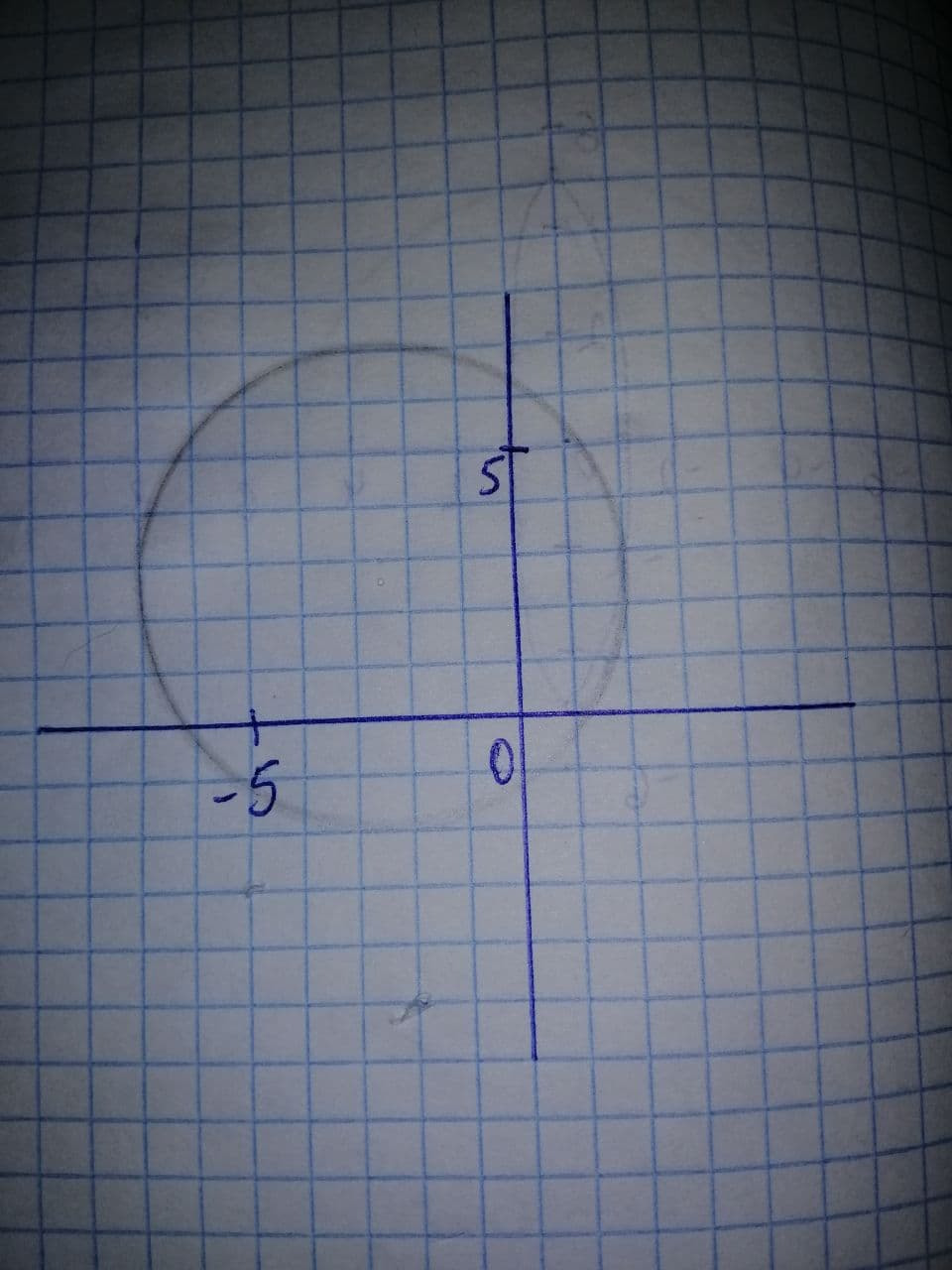Graph the equation x^{2}+y^{2}+4x-6y-3=0 in a rectangular coordinate system. IfEichowa4 2021-11-26 Answered
Graph the equation $$\displaystyle{x}^{{{2}}}+{y}^{{{2}}}+{4}{x}-{6}{y}-{3}={0}$$ in a rectangular coordinate system. If two functions are indicated, graph both in the same system.Then use your graphs to identify the relation’s domain and range.

• Questions are typically answered in as fast as 30 minutes

Solve your problem for the price of one coffee

• Math expert for every subject
• Pay only if we can solve itSusan Yang

Step 1
Given:
The equation $$\displaystyle{x}^{{{2}}}+{y}^{{{2}}}+{4}{x}-{6}{y}-{3}={0}$$
First using the software graph the function, and then by the analysis of the graph find the domain and range.It is clear from the graph that the domain of the function is $$\displaystyle-{6}{<}{x}{<}{2}$$
and the range of the graph of a function is $$\displaystyle-{1}{<}{y}{<}{7}$$